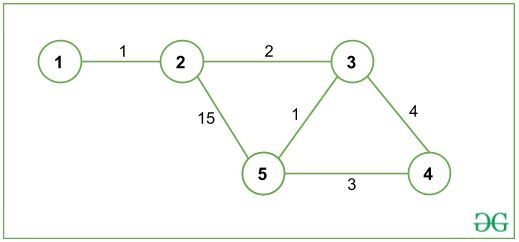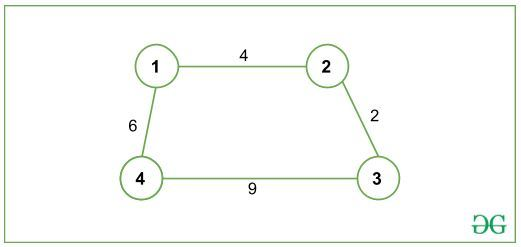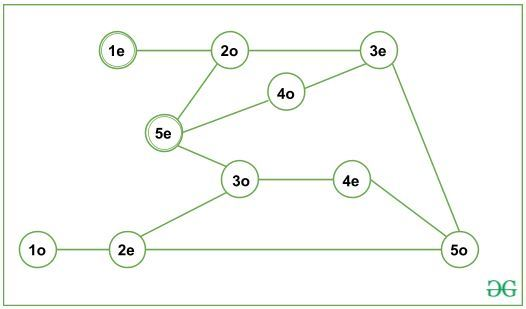# Shortest Path with even number of Edges from Source to Destination

• Last Updated : 19 Jan, 2022

Given an undirected graph G, the task is to find the shortest path of even-length, given 1 as Source Node and N as Destination Node. Path length refers to the number of edges present in a path (not the cost of the path).

Examples:

Input: N = 5, G is given below:Output: 10
Explanation:
All paths from 1(source node) to 5 (destination node) are:
1->2->5
Cost: 16 Length: 2(even)
1->2->3->5
Cost: 4 Length: 3(odd)
1->2->3->4->5
Cost: 10 Length: 4(even)
The shortest path is 1->2->3->5 with total cost 4, but it has an odd-length path and since we are interested in even-length paths only, the shortest path with even-length is 1->2->3->4->5, with total cost 10.

Input 2: N = 4, G is given below:Output: -1
Explanation:
There is no path of even-length from 1(source node) to 4(destination node).

Approach:
Create a new Graph (G’). For each node V in initial graph G, create two new nodes V_even and V_odd

Here, V_odd will be represented as ((V * 10) + 1) and V_even as ((V * 10) + 2).
For example, if node V = 4 then V_odd = 41 and V_even = 42.

Now, for each edge (U, V) in G, add two new edges in G’, (U_even, V_odd) and (U_odd, V_even). Finally, find the Shortest path from (source_even) node to (destination_even) node using Dijkstra Shortest Path Algorithm.
For Graph given in Input 1(above), G’ can be represented as:It can observed from the graph G’ that there are only even length paths from (1_even) to (5_even). Thus, the odd-length paths get separated in G’ and the required shortest path can be obtained.

Below is the implementation of the above approach:

## C++

 `// C++ program for the above approach``#include ``using` `namespace` `std;` `const` `int` `MAXX = 10000, INF = 1e9;` `// Adjacency List: to represent graph``vector > >``    ``adj(MAXX * 10 + 3);` `// Distance Array: to store shortest``// distance to every node``vector<``int``> dist(MAXX * 10 + 3, INF);` `// returns value which will``// represent even_x``int` `even(``int` `x)``{``    ``return` `x * 10 + 2;``}``// returns value which will``// represent odd_x``int` `odd(``int` `x)``{``    ``return` `x * 10 + 1;``}` `// converting edge (a->b) to 2``// different edges i.e. (a->b)``// converts to (1). even_a -> odd_b``// (2). odd_a -> even_b``// since, graph is undirected, so we``// push them in reverse order too``// hence, 4 push_back operations are``// there.``void` `addEdge(``int` `a, ``int` `b, ``int` `cost)``{``    ``adj[even(a)].push_back(``        ``{ odd(b), cost });``    ``adj[odd(a)].push_back(``        ``{ even(b), cost });``    ``adj[odd(b)].push_back(``        ``{ even(a), cost });``    ``adj[even(b)].push_back(``        ``{ odd(a), cost });``}` `// Function calculates shortest``// distance to all nodes from``// "source" using Dijkstra``// Shortest Path Algorithm``// and returns shortest distance``// to "destination"``int` `dijkstra(``int` `source,``             ``int` `destination)``{` `    ``/* Priority Queue/min-heap``    ``to store and process``    ``(distance, node) */``    ``priority_queue,``                   ``vector >,``                   ``greater > >``        ``pq;` `    ``// pushing source node to``    ``// priority queue and dist from``    ``// source to source is set to 0``    ``pq.push({ 0, even(source) });``    ``dist[even(source)] = 0;` `    ``while` `(!pq.empty()) {` `        ``// U is the node at top``        ``// of the priority queue``        ``// note that pq.top().first``        ``// refers to the Distance``        ``// and pq.top().second``        ``// will refer to the Node``        ``int` `u = pq.top().second;``        ``pq.pop();` `        ``// exploring all neighbours``        ``// of node u``        ``for` `(pair<``int``, ``int``> p :``             ``adj[u]) {` `            ``/* v is neighbour node of u``          ``and c is the cost/weight``          ``of edge (u, v) */``            ``int` `v = p.first;``            ``int` `c = p.second;` `            ``// relaxation: checking if there``            ``// is a shorter path to v via u``            ``if` `(dist[u] + c``                ``< dist[v]) {` `                ``// updating distance of v``                ``dist[v] = dist[u] + c;``                ``pq.push({ dist[v], v });``            ``}``        ``}``    ``}` `    ``// returning shortest``    ``// distance to "destination"``    ``return` `dist[even(destination)];``}` `// Driver function``int` `main()``{``    ``// n = number of Nodes,``    ``// m = number of Edges``    ``int` `n = 5, m = 6;``    ``addEdge(1, 2, 1);``    ``addEdge(2, 3, 2);``    ``addEdge(2, 5, 15);``    ``addEdge(3, 5, 1);``    ``addEdge(3, 4, 4);``    ``addEdge(5, 4, 3);` `    ``int` `source = 1;``    ``int` `destination = n;``    ``int` `ans = dijkstra(source, destination);` `    ``// if ans is INF: There is no``    ``// even length path from source``    ``// to destination else path``    ``// exists and we print the``    ``// shortest distance``    ``if` `(ans == INF)``        ``cout << ``"-1"``             ``<< ``"\n"``;``    ``else``        ``cout << ans << ``"\n"``;` `    ``return` `0;``}`

## Java

 `// Java program for the above approach``import` `java.util.ArrayList;``import` `java.util.Arrays;``import` `java.util.PriorityQueue;` `class` `GFG{` `static` `class` `Pair ``implements` `Comparable``{``    ``int` `first, second;` `    ``public` `Pair(``int` `first, ``int` `second)``    ``{``        ``this``.first = first;``        ``this``.second = second;``    ``}` `    ``@Override``    ``public` `int` `compareTo(GFG.Pair o)``    ``{``        ``if` `(``this``.first == o.first)``        ``{``            ``return` `this``.second - o.second;``        ``}``        ``return` `this``.first - o.first;``    ``}``}` `static` `final` `int` `MAXX = ``10000``, INF = (``int``)1e9;` `// Adjacency List: to represent graph``@SuppressWarnings``(``"unchecked"``)``static` `ArrayList[] adj = ``new` `ArrayList[MAXX * ``10` `+ ``3``];` `// Distance Array: to store shortest``// distance to every node``static` `int``[] dist = ``new` `int``[MAXX * ``10` `+ ``3``];` `// Returns value which will``// represent even_x``static` `int` `even(``int` `x)``{``    ``return` `x * ``10` `+ ``2``;``}` `// Returns value which will``// represent odd_x``static` `int` `odd(``int` `x)``{``    ``return` `x * ``10` `+ ``1``;``}` `// Converting edge (a->b) to 2``// different edges i.e. (a->b)``// converts to (1). even_a -> odd_b``// (2). odd_a -> even_b``// since, graph is undirected, so we``// push them in reverse order too``// hence, 4 push_back operations are``// there.``static` `void` `addEdge(``int` `a, ``int` `b, ``int` `cost)``{``    ``adj[even(a)].add(``new` `Pair(odd(b), cost));``    ``adj[odd(a)].add(``new` `Pair(even(b), cost));``    ``adj[odd(b)].add(``new` `Pair(even(a), cost));``    ``adj[even(b)].add(``new` `Pair(odd(a), cost));``}` `// Function calculates shortest``// distance to all nodes from``// "source" using Dijkstra``// Shortest Path Algorithm``// and returns shortest distance``// to "destination"``static` `int` `dijkstra(``int` `source, ``int` `destination)``{``    ` `    ``// Priority Queue/min-heap to store``    ``// and process (distance, node)``    ``PriorityQueue pq = ``new` `PriorityQueue<>();` `    ``// Pushing source node to``    ``// priority queue and dist from``    ``// source to source is set to 0``    ``pq.add(``new` `Pair(``0``, even(source)));``    ``dist[even(source)] = ``0``;` `    ``while` `(!pq.isEmpty())``    ``{``        ` `        ``// U is the node at top``        ``// of the priority queue``        ``// note that pq.top().first``        ``// refers to the Distance``        ``// and pq.top().second``        ``// will refer to the Node``        ``int` `u = pq.poll().second;` `        ``// Exploring all neighbours``        ``// of node u``        ``for``(Pair p : adj[u])``        ``{``            ` `            ``// v is neighbour node of u and``            ``// c is the cost/weight of edge (u, v)``            ``int` `v = p.first;``            ``int` `c = p.second;` `            ``// Relaxation: checking if there``            ``// is a shorter path to v via u``            ``if` `(dist[u] + c < dist[v])``            ``{``                ` `                ``// Updating distance of v``                ``dist[v] = dist[u] + c;``                ``pq.add(``new` `Pair(dist[v], v));``            ``}``        ``}``    ``}``    ` `    ``// Returning shortest``    ``// distance to "destination"``    ``return` `dist[even(destination)];``}` `// Driver code``public` `static` `void` `main(String[] args)``{``    ``for``(``int` `i = ``0``; i < MAXX * ``10` `+ ``3``; i++)``    ``{``        ``adj[i] = ``new` `ArrayList();``    ``}` `    ``Arrays.fill(dist, INF);` `    ``// n = number of Nodes,``    ``// m = number of Edges``    ``int` `n = ``5``, m = ``6``;``    ``addEdge(``1``, ``2``, ``1``);``    ``addEdge(``2``, ``3``, ``2``);``    ``addEdge(``2``, ``5``, ``15``);``    ``addEdge(``3``, ``5``, ``1``);``    ``addEdge(``3``, ``4``, ``4``);``    ``addEdge(``5``, ``4``, ``3``);` `    ``int` `source = ``1``;``    ``int` `destination = n;``    ``int` `ans = dijkstra(source, destination);` `    ``// If ans is INF: There is no``    ``// even length path from source``    ``// to destination else path``    ``// exists and we print the``    ``// shortest distance``    ``if` `(ans == INF)``        ``System.out.println(``"-1"``);``    ``else``        ``System.out.println(ans);``}``}` `// This code is contributed by sanjeev2552`

## Python3

 `# Python3 program for the above approach``import` `heapq as hq` `MAXX ``=` `10000``INF ``=` `1e9` `# Adjacency List: to represent graph``adj ``=` `[[] ``for` `_ ``in` `range``(MAXX ``*` `10` `+` `3``)]` `# Distance Array: to store shortest``# distance to every node``dist ``=` `[INF] ``*` `(MAXX ``*` `10` `+` `3``)` `# returns value which will``# represent even_x``def` `even(x):``    ``return` `x ``*` `10` `+` `2`  `# returns value which will``# represent odd_x``def` `odd(x):``    ``return` `x ``*` `10` `+` `1`  `# converting edge (a->b) to 2``# different edges i.e. (a->b)``# converts to (1). even_a -> odd_b``# (2). odd_a -> even_b``# since, graph is undirected, so we``# push them in reverse order too``# hence, 4 append operations are``# there.``def` `addEdge(a, b, cost):``    ``adj[even(a)].append((odd(b), cost))``    ``adj[odd(a)].append((even(b), cost))``    ``adj[odd(b)].append((even(a), cost))``    ``adj[even(b)].append((odd(a), cost))`  `# Function calculates shortest``# distance to all nodes from``# "source" using Dijkstra``# Shortest Path Algorithm``# and returns shortest distance``# to "destination"``def` `dijkstra(source, destination):` `    ``# Priority Queue/min-heap``    ``# to store and process``    ``# (distance, node)``    ``pq ``=` `[]` `    ``# pushing source node to``    ``# priority queue and dist from``    ``# source to source is set to 0``    ``hq.heappush(pq, (``0``, even(source)))``    ``dist[even(source)] ``=` `0` `    ``while` `pq:` `        ``# U is the node at top``        ``# of the priority queue``        ``# note that pq.top()``        ``# refers to the Distance``        ``# and pq.top()``        ``# will refer to the Node``        ``u ``=` `hq.heappop(pq)[``1``]` `        ``# exploring all neighbours``        ``# of node u``        ``# v is neighbour node of u``        ``# and c is the cost/weight``        ``# of edge (u, v)``        ``for` `v, c ``in` `adj[u]:` `            ``# relaxation: checking if there``            ``# is a shorter path to v via u``            ``if` `dist[u] ``+` `c < dist[v]:` `                ``# updating distance of v``                ``dist[v] ``=` `dist[u] ``+` `c``                ``hq.heappush(pq, (dist[v], v))` `    ``# returning shortest``    ``# distance to "destination"``    ``return` `dist[even(destination)]`  `# Driver function``if` `__name__ ``=``=` `"__main__"``:``    ``# n = number of Nodes,``    ``# m = number of Edges``    ``n ``=` `5``    ``m ``=` `6``    ``addEdge(``1``, ``2``, ``1``)``    ``addEdge(``2``, ``3``, ``2``)``    ``addEdge(``2``, ``5``, ``15``)``    ``addEdge(``3``, ``5``, ``1``)``    ``addEdge(``3``, ``4``, ``4``)``    ``addEdge(``5``, ``4``, ``3``)` `    ``source ``=` `1``    ``destination ``=` `n``    ``ans ``=` `dijkstra(source, destination)` `    ``# if ans is INF: There is no``    ``# even length path from source``    ``# to destination else path``    ``# exists and we print``    ``# shortest distance``    ``if` `ans ``=``=` `INF:``        ``print``(``-``1``)``    ``else``:``        ``print``(ans)`

Output:

`10`

Time Complexity: (E * log(V))
Auxiliary Space: O(V + E)

My Personal Notes arrow_drop_up